### Signals and systems - Part B – Important Questions

##### UNIT I

1. Determine whether the following systems are linear,time invariant,causal ,stable.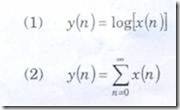2. Determine whether the following systems are linear or not

dy(t) / dt + 3ty(t) = t2x(t) & y(n)=2x(n)+ 1 / x(n-1)

3. Explain the classification of signals with examples

4. Determine whether the following systems are Time-Invarient or not

Y(t) = t x(t) & y(n) = x(2n)

5. (a) Find whether the signal x(t) = 2 cos (10 t+1) – sin(4t-1) is periodic or not.

(b) Evaluate Σ n=( -∞ to ∞) e2n δ (n-2)

(c) Find the fundamental period of the Continuous time signal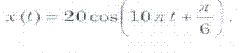#### UNIT II

1. Find the inverse laplace transform of X(S) = S / S2+5S+6

2. Find the fourier transform of a rectangular pulse of duration T and amplitude A

3. Obtain the cosine fourier series representation of x(t)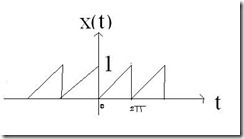4. Find the trigonometric fourier series of the figure shown below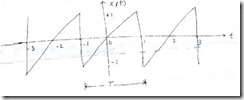5. Find the laplace transform of the signal x (t) = eat u(t) + e bt u(t)

##### UNIT III

1. Find the convolution of the two signals x(t)= e -2t u(t) h(t)= u(t+2)

2. State and prove the convolution property of Z-Transform

3. Determine the Z=Transform of x1(n)=an and x2(n) =nu(n)

4. Find the convolution of x(t) = u(t+1) and h(t) = u(t-2)

5. Find the Fourier transform of x(t) = t cos ωt

##### UNIT IV

1. Find the Unilateral Z-transform and R.O.C of x(n) = sin ω0 n u(n)

2. Discuss the block diagram representation of an LTI-DT system

3. Consider a causal LTI system as in the fig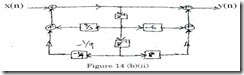Determine the differential equation relating x(n) and y(n).

4. State and prove the Parseval’s relation.

5. Explain any 4 properties of DTFT.

##### UNIT V

1. Develop the Direct form I & II realization of the differential equation

dy(t) / dt + 5 x(t) = 3 x(t)

2. Prove any 2 properties of Z-transform

3. Obtain the cascade form realization of the system described by the differential Equation

y(n) – ¼ y (n-1) – 1/8 y (n-2) = x(n) + 3 x(n-1) +2 x(n-2)

4. Find the state variable matrices A,B,C,D for the equation

y(n) - 3y(n-1) - 2y(n-2) = x(n) + 5 x(n-1) + 6 x(n-2)

5. Discuss the block diagram representation of an LTI-DT system